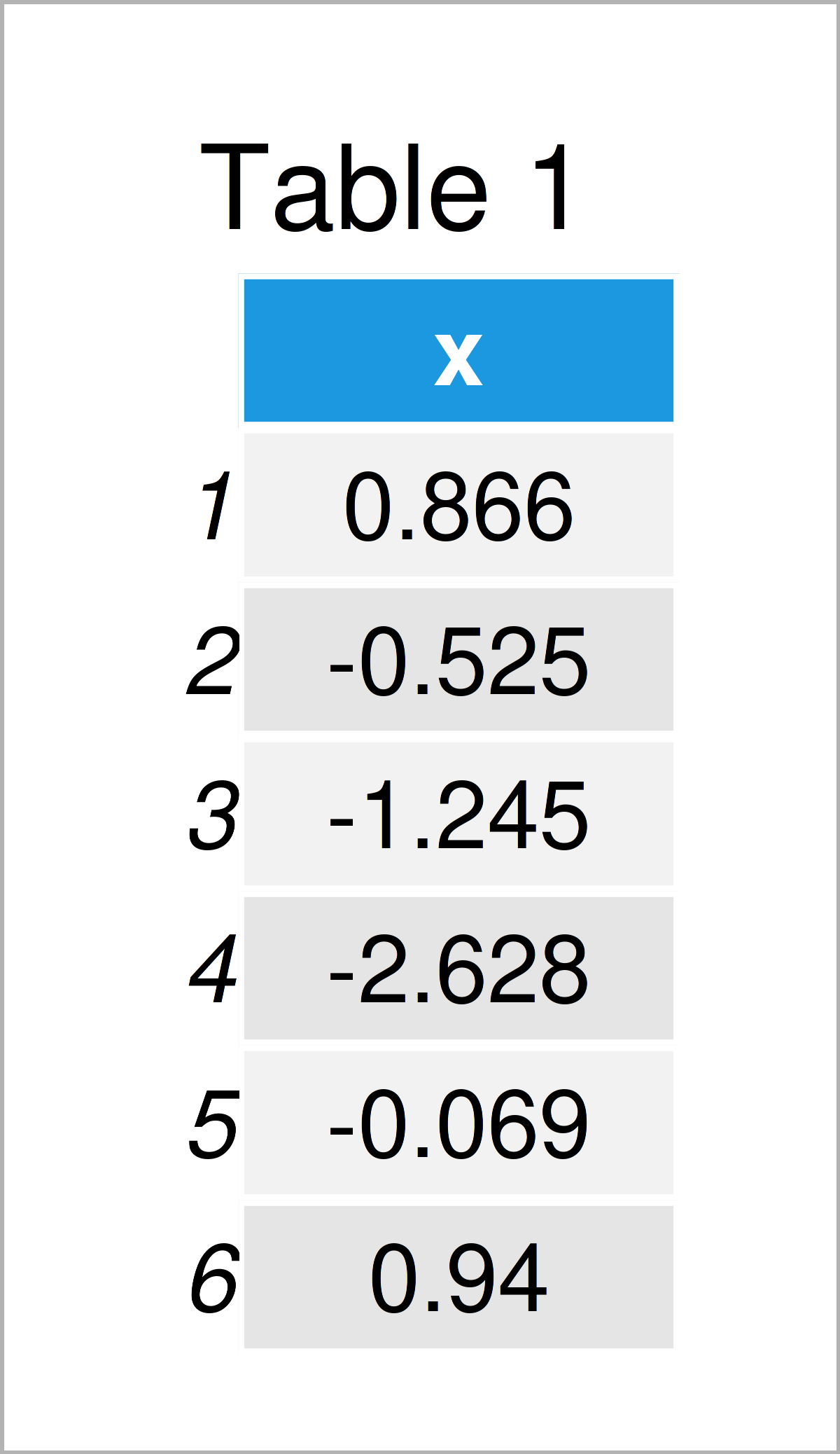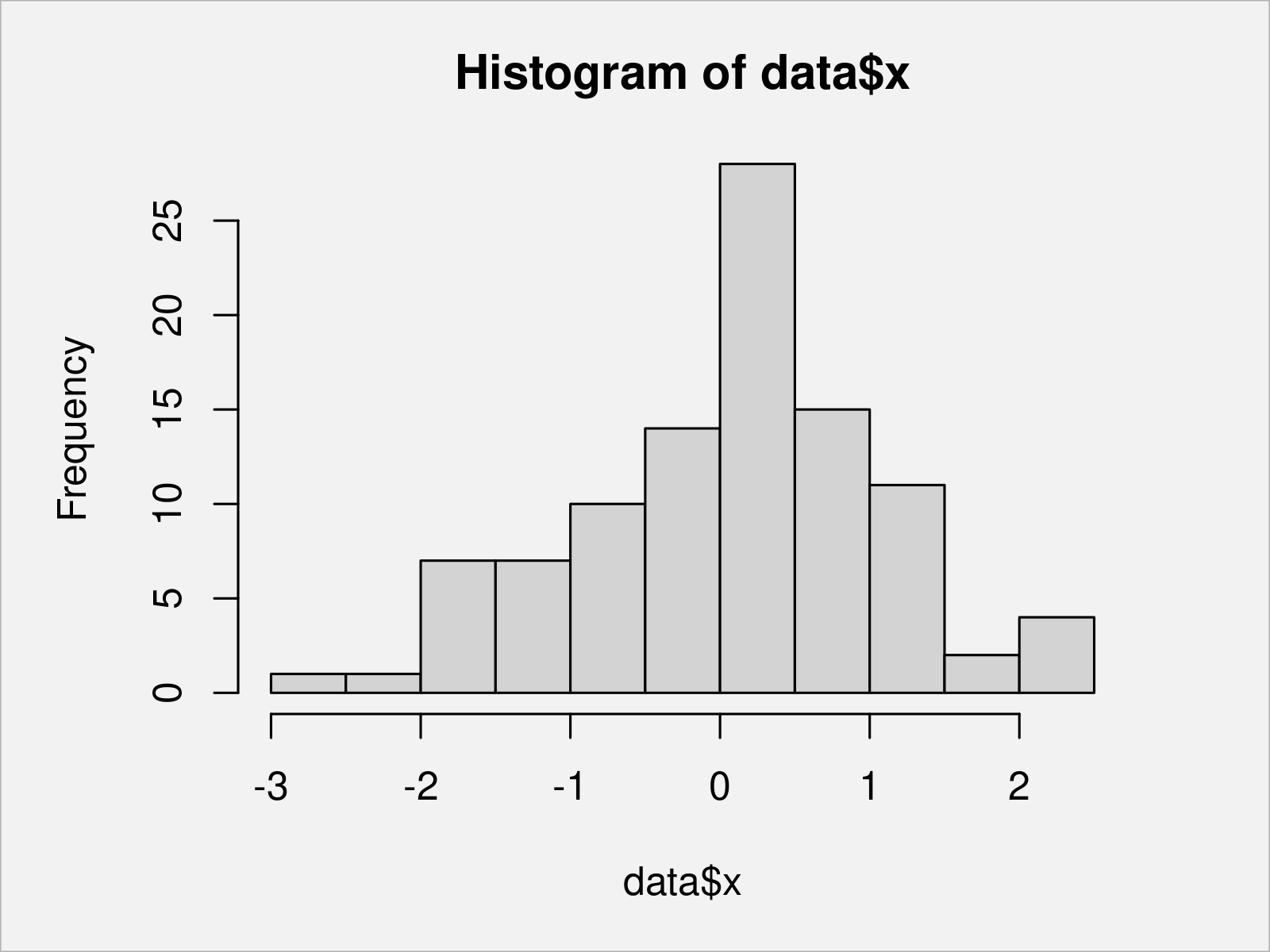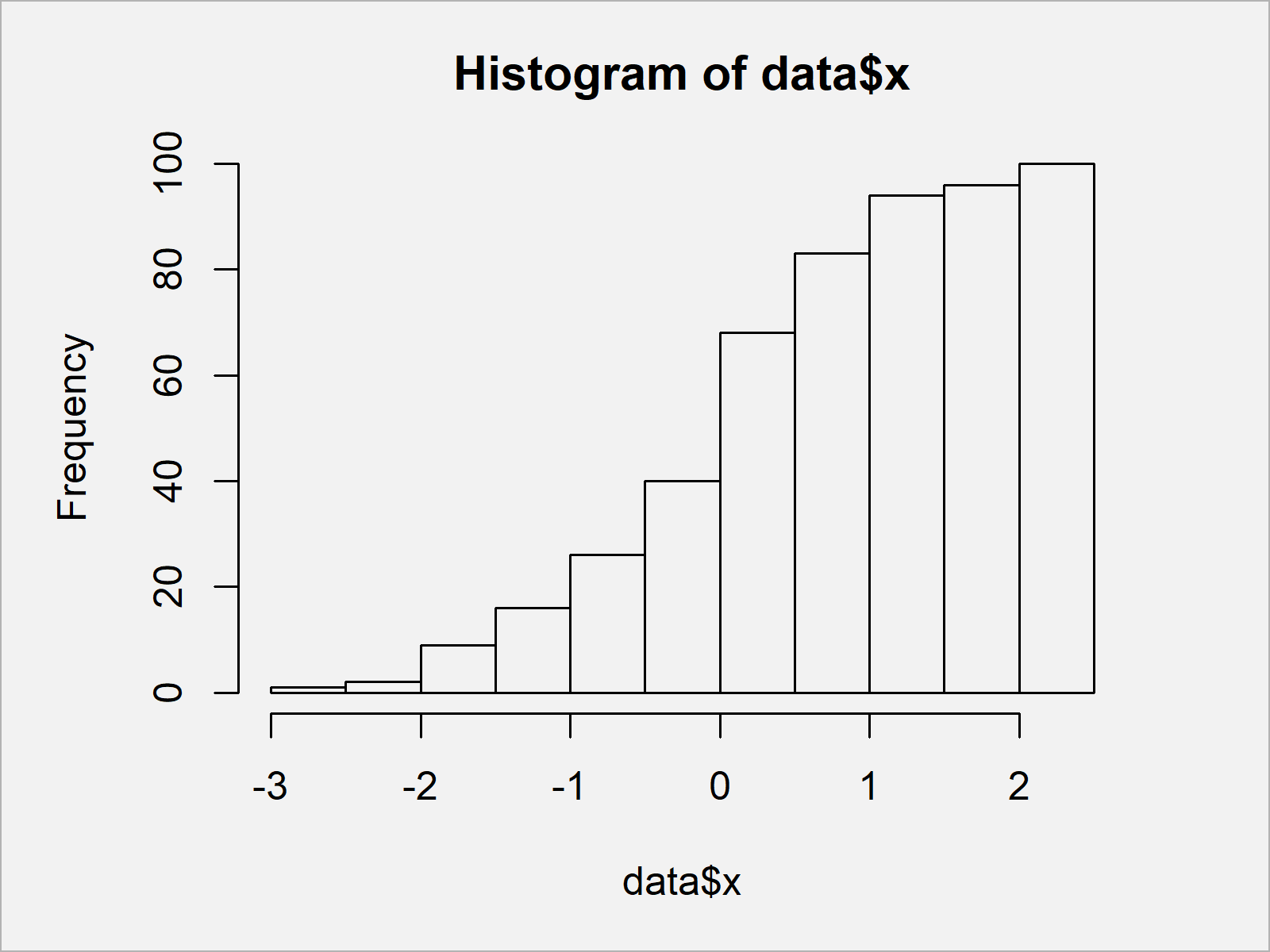# Draw Cumulative Histogram in R (2 Examples) | Base R & ggplot2 Package

In this R tutorial you’ll learn how to draw the cumulative sum of a vector in a histogram.

Here’s how to do it!

## Example Data

The following data is used as basement for this R programming language tutorial:

```set.seed(258967)                            # Create example data
data <- data.frame(x = rnorm(100))Table 1 illustrates the output of the RStudio console that has been returned after executing the previous syntax and shows the head of our example data. Furthermore, it is shown that our data is constituted of only one column.

## Example 1: Plot Cumulative Histogram Using Base R

In Example 1, I’ll demonstrate how to draw a cumulative frequency histogram using the basic installation of the R programming language.

Let’s first draw a histogram with default specifications:

`hist(data\$x)                                # Draw default histogram`In Figure 1 it is shown that we have plotted a Base R histogram by executing the previously shown R programming syntax.

If we want to create a histogram showing the cumulative sum of our data, we can use the R code below. In this R code, we use the hist function to extract and modify the bars of our histogram:

```my_hist <- hist(data\$x)                     # Store histogram info
my_hist\$counts <- cumsum(my_hist\$counts)    # Change histogram counts
plot(my_hist)                               # Draw cumulative histogram```Figure 2 visualizes our data in a cumulative histogram. Note that we could also use different cumulative distributions such as the cumulative mean, the cumulative maxima, or the cumulative minima to draw our histogram.

## Example 2: Plot Cumulative Histogram Using ggplot2 Package

In Example 2, I’ll show how to plot a cumulative histogram using the ggplot2 package.

In order to use the functions of the ggplot2 package, we first need to install and load ggplot2:

```install.packages("ggplot2")                 # Install ggplot2 package

Next, we can draw a ggplot2 histogram with default specifications as shown below:

```ggplot(data, aes(x)) +                      # Draw default ggplot2 histogram
geom_histogram()```As shown in Figure 3, we have created a ggplot2 histogram without cumulative values.

If we want to convert our histogram to a cumulative histogram, we can use the cumsum function within the geom_histogram function as shown below:

```ggplot(data, aes(x)) +                      # Draw cumulative ggplot2 histogram
geom_histogram(aes(y = cumsum(..count..)))```Figure 4 reveals the output of the previous R programming code: A cumulative ggplot2 histogram.

## Video & Further Resources

Would you like to know more about the drawing of the cumulative sum in a histogram? Then I recommend watching the following video which I have published on my YouTube channel. I show the R code of this tutorial in the video:

Please accept YouTube cookies to play this video. By accepting you will be accessing content from YouTube, a service provided by an external third party.If you accept this notice, your choice will be saved and the page will refresh.

In addition, you might read the other tutorials which I have published on this homepage. I have released several articles already:

This article has demonstrated how to plot the cumulative sum in a histogram in the R programming language. If you have additional questions, let me know in the comments.

Subscribe to the Statistics Globe Newsletter